# Anatomy of An Idiom

### Introduction

Sometime in the early 1970s while I was working at STSC, Jeff Lewis, then a customer of ours at The Rouse Company, posed a challenging APL problem I named Progressive Dyadic Iota: devise an algorithm to find the index of each element of one vector in another where each successive element of the right argument should match the corresponding successive element of the left argument.

### An Example

For example, if the left and right arguments were 'abacba' and 'baabaac', then the result would be

L'abacba' R'baabaac'
L pdi R
2 1 3 5 6 7 4

Notice how the first b in R matches the first b in L at index 2 and that the second b in R matches the second b in L at index 5, etc.

### Labeling

In other words, it was as if the elements of the left and right arguments had been labeled with subscripts indicating the successive positions of equal values:

'a1b1a2c1b2a3' 'b1a1a2b2a3a4c1'
2 1 3 5 6 7 4

### Ranking To The Rescue

Labeling the successive equal elements of a vector as above is a job for the ranking function (i.e., ), as in

L,[0.5] LL
a b a c b a
1 4 2 6 5 3

which labels the 'a's as 1 2 3, the 'b's as 4 5, and the lone 'c' as 6.

Applying this technique to both arguments and then looking up the right in the left solves the problem if the arguments satisfy several conditions:

1. The arguments contain the same set of elements,
2. The number of elements of each value is the same in both arguments, and
3. The unique elements of both arguments occur in the same order
L'abacba' R'aaabcb'
L pdi R
1 3 6 2 4 5
(LL)RR
1 3 6 2 4 5

### Lookup Left

With a little thought, it's easy to see that the third condition can be eliminated by using the left argument as the lookup vector on both sides:

L'abacba' R'bcabaa'
L(LL),[0.5] LL
a b a c b a
1 2 1 4 2 1
1 4 2 6 5 3
R(LR),[0.5] LR
b c a b a a
2 4 1 2 1 1
4 6 1 5 2 3
L pdi R
2 4 1 5 3 6
(LL)LR
2 4 1 5 3 6

### A Key Concept

Next, we need a way to equalize the numbering on both sides. That is, the 'a's are already numbered the same on both sides, but the 'b's are not necessarily because there might be more 'a's in L than in R. This can be solved by including R in the lefthand double grade-up and similarly including L on the righthand side. In other words,

(LL,R)LR,L
2 4 1 5 3 6 9 7 11 8 10 12

Notice how this simple but important step of including the other argument on each side's lookup and ranking each lookup provides just the right balance. Not incidentally, the algorithm so far is now independent of the number of elements of each value in both arguments, thus eliminating the second condition.

### Shaping Up

Since we need only the first R elements of this expression for the result, we can reshape the righthand side of the middle iota, as in

(LL,R)(R)LR,L
2 4 1 5 3 6

With the algorithm so far, if an element of R is not found in L, it'll be assigned a value of IO+(L)+R, but we actually need IO+L. This can be solved by reshaping the lefthand side of the middle iota to the same shape as L, as in

L'abacba' R'bcdabaa'
((L)LL,R)(R)LR,L
2 4 7 1 5 3 6

thus eliminating the last remaining condition.

### All Together Now

Thus, each argument to the middle iota is a ranking of the respective argument in L such that equal values in the two arguments have the same ranking on each side. It is this equal ranking which allows the middle iota to produce the correct result.

In other words, what we have done is similar to the labeling described above, but the indices are now sequential depending upon first the total number of 'a's, then the total number of 'b's, etc.

In the example below, there are seven 'a's between the two arguments, so the 'b's begin numbering with eight, even though the 'a's are numbered only from one to three in L and one to four in R. Continuing, there are four 'b's between the two arguments, so the 'c's begin numbering with twelve (the 'b's start at eight plus four of them).

Also, note that a4 in R is not found in L, so it is assigned an index of seven (i.e., IO+L).

L'abacba' R'baabaac'
L pdi R
2 1 3 5 6 7 4
'a1b8a2c12b9a3' 'b8a1a2b9a3a4c12' (†)
2 1 3 5 6 7 4
(L)LL,R
1 8 2 12 9 3
(R)LR,L
8 1 2 9 3 4 12

Note that the two sets of subscripts in statement (†) are exactly the left and right arguments to the middle iota. That is, the subscripts are unique such that we can dispense with the letters and just use the subscripts which is exactly what is done.

Here's the final function:

ZL pdi R
   Z((L)LL,R)(R)LR,L

### A Related Function

As Dyadic Iota and Epsilon are closely related, it should come as no surprise that there is a corresponding Progressive Dyadic Epsilon.

ZL pde R
   Z((L)LL,R)(R)LR,L

That is, pdi and pde differ in their principal (and middle) function, only.

This latter function has some interesting uses such as in calculating an Asymmetric Difference between two multisets, where a multiset is a set (i.e. vector) with repeated elements. Asymmetric difference is like set difference, but where the multiplicity of each unique element in the left argument has subtracted from it the multiplicity of the same valued element in the right argument. If the difference between the multiplicities is positive, then that many copies of that element appear in the result. For multisets with no repeated elements, asymmetric difference and set difference produce the same results.

For example,

    Multiset Asymmetric Difference
   Z(~L pde R)/L

L6 1 2 3 3 3 4 4 4 2 6 4
R1 1 1 1 2 3 3 4 5 5 5
6 3 4 4 2 6 4

### Citations

• You might remember this idiom because, purely by accident, it appears at the very top of the Finn APL Idiom List.
• Or this Unicode version of the same Finn APL Idiom List.
• Also see J's approach.
• This idiom was published in APL Quote-Quad, Volume 4, Issue 1, September 1972 as the solution to Problem #14 in my Problems section (thanks to Gary Logan for the reference).

### Fonts

If you have trouble displaying the APL characters on this page, likely it is due to either a browser setting (or an out-of-date browser) or a missing font. Both Mozilla Firefox 2.0 or later and Internet Explorer 7 or later display the APL characters perfectly, but IE6 has some trouble. If this page doesn't display well with either APL Unicode font using any version of Internet Explorer, please try it again with Mozilla Firefox. Links for the two APL Unicode fonts as well as for Mozilla Firefox appear at the top of this page.

### Author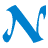NARS2000© 2006-2020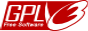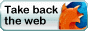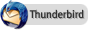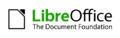Comments or suggestions? Send them to .